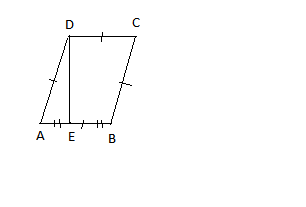# Problem from IMOABCD is a rhombus in which the altitude from d bisects AB. AE=EB. Therefore, angle A and angle B respectively are (of how many degrees).7 years, 9 months ago

This discussion board is a place to discuss our Daily Challenges and the math and science related to those challenges. Explanations are more than just a solution — they should explain the steps and thinking strategies that you used to obtain the solution. Comments should further the discussion of math and science.

When posting on Brilliant:

• Use the emojis to react to an explanation, whether you're congratulating a job well done , or just really confused .
• Ask specific questions about the challenge or the steps in somebody's explanation. Well-posed questions can add a lot to the discussion, but posting "I don't understand!" doesn't help anyone.
• Try to contribute something new to the discussion, whether it is an extension, generalization or other idea related to the challenge.

MarkdownAppears as
*italics* or _italics_ italics
**bold** or __bold__ bold
- bulleted- list
• bulleted
• list
1. numbered2. list
1. numbered
2. list
Note: you must add a full line of space before and after lists for them to show up correctly
paragraph 1paragraph 2

paragraph 1

paragraph 2

[example link](https://brilliant.org)example link
> This is a quote
This is a quote
    # I indented these lines
# 4 spaces, and now they show
# up as a code block.

print "hello world"
# I indented these lines
# 4 spaces, and now they show
# up as a code block.

print "hello world"
MathAppears as
Remember to wrap math in $$ ... $$ or $ ... $ to ensure proper formatting.
2 \times 3 $2 \times 3$
2^{34} $2^{34}$
a_{i-1} $a_{i-1}$
\frac{2}{3} $\frac{2}{3}$
\sqrt{2} $\sqrt{2}$
\sum_{i=1}^3 $\sum_{i=1}^3$
\sin \theta $\sin \theta$
\boxed{123} $\boxed{123}$

Sort by:

I don't think this is from IMO (International Mathematical Olympiad).

- 7 years, 9 months ago

This is from an exam conducted here in India by a private company.

- 7 years, 9 months ago

Oh, I see. Indian Mathematical Olympiad?

- 7 years, 9 months ago

No way, I doubt they ask such easy questions. As Vikram said, it must be a contest by some private organisation.

If the private organisations use names such as "IMO" and mislead the students, then its a very bad tactic to promote themselves.

- 7 years, 9 months ago

its called international mathematics olympiad conducted by SOF

- 7 years, 9 months ago

Nope. Its a basic level contest conducted by a company.

- 7 years, 9 months ago

Hey guys,I think she is talking of SOF IMO and not the Great and the one you all are thinking IMO!!!!

- 7 years, 9 months ago

YOU,RE RIGHT!. It is a problem from the work book.

- 7 years, 9 months ago

Consider the sides of the rhombus to be of length $x$, i.e., $AD=x$. So, $AE= \frac{x}{2}$.

Let $\angle A= \theta$. So, $cos \theta = \frac{AE}{AD} =\frac{1}{2}$

$\implies \theta =60^o$ $\implies \angle A=60^o$ and $\angle B=180^o-60^o=120^o$(As they are interior opposite angles)

- 7 years, 9 months ago

This que is not from IMO !

- 7 years, 9 months ago

I think is 180 degrees

- 7 years, 9 months ago

Consider- $DA = x$

$AE = x/2$

$EB = x/2$

$DE = x^{2} - (x/2)^{2}$ $= \sqrt{3}x/2$

$DB = x$ (Pythagoras Theorem)

$DA = x , DB = x , AB = x$

$ADB$ is an Equilateral triangle

Thus, $A = 60°$ $B = 120°$

- 7 years, 9 months ago

THANK YOU!!!

- 7 years, 9 months ago

ANGLE A=60 ANGLE B=120

- 7 years, 9 months ago

If this problem is from IMO, please tell me what year and what question.

- 7 years, 9 months ago

- 7 years, 9 months ago

this problem is not from the main exam, it is from the workbook

- 7 years, 9 months ago